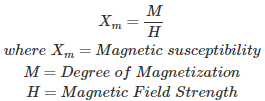# MCQs on Electric Circuits

##### Page 4 of 63. Go to page 1 2 3 4 5 6 7 8 9 10 11 12 13 14 15 16 17 18 19 20 21 22 23 24 25 26 27 28 29 30 31 32 33 34 35 36 37 38 39 40 41 42 43 44 45 46 47 48 49 50 51 52 53 54 55 56 57 58 59 60 61 62 63
01․ Which of the following has no units?
Permeability
Moment of a magnet
Magnetic susceptibility
Permittivity

Magnetic susceptibility is the degree to which a material is magnetized by an external magnetic field, hence in the SI system it is dimensionless.02․ Which of the following quantities consists of SI unit as WATT ?
Force
Charge
Current
Power

The SI unit of power is the watt and it is discover by the Scottish Engineer James Watt. Power is the rate of consumption of electric energy. So, it is equivalent to joules per second.

03․ KCL works on the principle of which of the following
law of conservation of charge.
law of conservation of energy.
both.
None of the above.

KCL (Kirchhoff's Current Law) states that in an electrical circuit total current entering in to a node is equal to the total current leaving from node. This works on the principle of law of conservation of charge. As the current is rate flow of charge. So, charge is conserved at a node.

04․ KVL works on the principle of
law of conservation of charge.
law of conservation of energy.
both.
None of the above.

KVL (Kirchhoff’s Voltage Law) states that algebraic sum of voltages in a loop is zero. This works on the principle law of conservation of energy. As the voltage is analogous to potential and this law states that total potential gain and total potential drops along a loop are zero.

05․ Super mesh analysis is used in case of
current source branch is common for two meshes.
ideal voltage source is connected between two non reference nodes.
both.
either 1 or 2.

When an ideal or dependent current source branch is common for two meshes it is possible to find the solution by using super mesh analysis. Super mesh analysis is a better technique instead of using mesh analysis to analyze such a complex circuit or network where two meshes have a current source as a common element. In super mesh analysis technique, the current source is in the inner area of the super mesh. Therefore, we are able to reduce the number of meshes by one for each current source which is present in the network. Therefore in this case we are using super mesh analysis.

06․ When we use super node technique
current source branch is common for two meshes.
ideal voltage source is connected between two non reference nodes.
ideal voltage source is connected between non reference node and reference.
All of the above.

When ideal voltage source or dependent voltage source is connected between two non reference node, then it not is possible to find the solution by using node analysis technique. Then we apply supernode analysis technique.

07․ Rms value is defined based on which of the following?
Heating effect
Charge transfer
Current
Voltage

Rms (Root mean square) value is defined based on heating effect of wave-forms. The value at which heat dissipated in AC circuit is same as the heat dissipated in DC circuit is called rms value, provide both AC and DC circuits have equal value of resistance and operated for same time.

08․ Which of the following defined the average value ?
Voltage
Heating effect
Current
Charge transfer

Average value is defined based on charge transfer in the circuit. The voltage at which charge transfer in AC circuit is equal to charge transfer in the DC circuit is called as average value of the AC, provided both AC and DC circuits have equal value of resistance and operated for same time.

09․ For symmetrical wave form average value of one full cycle is
1
1.11
2.22
0

For symmetrical wave form both positive half cycle and negative half cycles are same. Therefore while finding average value these two half cycles gets cancelled out, so average value for symmetrical wave form is zero. Actually integrating over a full cycle from 0 to 2π is zero.

10․ Form factor is equal to Peak factor in case of
square wave.
triangle wave.
saw tooth wave.
all of the above.

The form factor of an alternating current waveform is the ratio of the rms value to the average value. The peak factor is the peak amplitude of the waveform divided by the rms value of the waveform. For square waveform form factor and peak factor is same and value is one.

<<<23456>>>